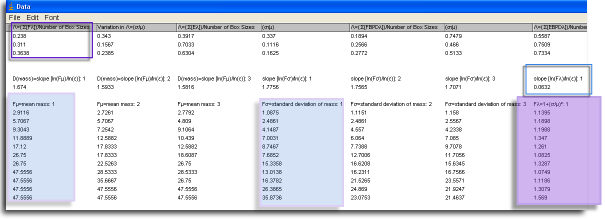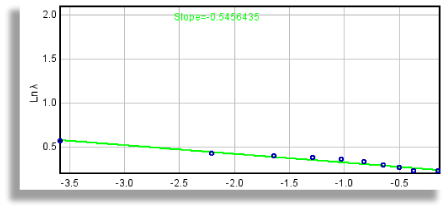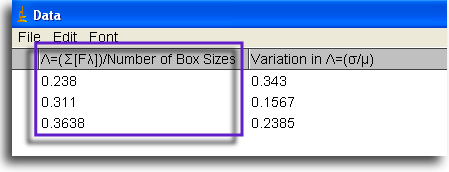Lacunarity is reported in several ways in FracLac.

Interpreting Lacunarity

Lacunarity is heterogeneity, a concept explained and, better yet, illustrated in the background section for lacunarity. How do the numbers that FracLac returns reflect the concept?

### First things First

We need to get one thing out of the way up front here. You have to know where to get the numbers for lacunarity that are discussed on this page. If you arrived here from a section on interpreting results in general, you already know that you get them from a Standard Box Count Scan, Sub Scan, Multifractal Scan, or a Sliding Box Lacunarity scan. Lacunarity results are reported in the various graphics, results files (e.g., the FracLac Results file, which appears with Standard Box Count scans), and data files (e.g., the Standard Box Count Data file, which appears only if the "Show Data" option is selected when you scan). Results from the SLAC scan we can talk about here.

### Per Box Size

#### What information can you get about lacunarity from these files?

Lacunarity is generally based on the pixel distribution for an image, which we get from scans at different box sizes at different grid orientations:

1. The most basic number for lacunarity, λ, is λε,g = CV2ε,g =(σ⁄μ)2ε,g where σ is the standard deviation and μ is the mean for pixels per box at this size, ε, in a box count at this orientation, g. To locate λ, look in the Data file where it is recorded per ε and per g.1. Because λ∝ε, there are many λs to deal with in the data file. λs and any other data from a box count are in sets of columns for each grid, g, in the lower part of the file. Each g has a column for each value, with a row for each ε. So, if there were 3 gs, you will see 3 columns of, for instance, σ labeled as σ: 1, σ: 2, and σ: 3, with rows corresponding to each ε listed at the far left of the file (but not shown in this image).
1. Individual λs are in columns labeled "Fλ =1+(σ/μ)2" (this is the same as Fλ=1+λ) The F stands for Foreground pixels, and signifies lacunarity calculated using the count of foreground pixels independently of other considerations.
In this regard, you may have noticed column headings that look like variations on Fλ in the data file. These are treated essentially the same as Fλ, and the same general ideas apply to finding and interpreting them; the differences lie in how the data were treated. That is, the calculations were made from a binned probability distribution for values with BPD in their names, and taking into account empty boxes encountered in a scan for values with E in their names. You can read their definitions in the glossary using these links:
1. Binned Probability Density Lacunarity (FBPD and EBPD)
• You can see where to find the data in the screen shot. The first g's Fλ=1+λ column is highlighted in purple, and its σ and μ columns in blue. (If you are wondering, the purple outline at the top left is discussed below in a zoomed in version of the file.)

### Over All Sizes

1. All of the λs from looking at our image using one orientation of a series of grid calibres can be summarized in two ways, as a slope and as a mean.
1. We can look at the slope of the ln-ln regression line of over all ε.
• To locate the slope in the data file, see the screen shot. The slope is boxed in a blue outline at the far right of the screen shot, which corresponds in the file itself to being listed above each column for Fλ=1+λ for each g.
• To see how λ∝ε graphically, before scanning, select Graph Lacunarity to generate graphs of the data and the regression line labeled with the slope.• Why use Fλ= 1+(σμ) 2=1+λ? The slope for each g is calculated using this transformation because of an "undefined" calculation. A completely homogeneous image will not vary in the pixels per box, so that the standard deviation, σ, for a box count at some ε will be 0. This means that λ = (σμ) 2 = 0, which makes sense, but it also means that the ln of λ and therefore the slope of the ln-ln regression line for λ and ε would be undefined. Thus, we transform the data using λ+1, so that a completely homogeneous image has a slope of 0, corresponding intuitively to the idea of no rotational or translational invariance and no gaps.
2. To put heterogeneity from one perspective and one series of grid sizes into an average, the mean (λ or Λ) from all ε sized boxes at a grid orientation, g, is calculated
• Λg = [Box SizesΣ b=1λε (b)] ÷ Box Sizes.
• To locate this summary value in the Data file, check the top rows. Find the grid orientation you want from the first column, then follow that row to the column heading for the particular type of Λ you need, in this case "F" at the top part of the data file (see Λ in the screen shot of part of a data file, outlined in purple).. Beside that value you can find the CV2, also, which describes some of the relative variation in Fλ over all ε. FracLac does not calculate the mode and median but these can be found readily by importing the data file into a spreadsheet and calculating these from the appropriate columns.

### Over All Orientations

1. To put all of your heterogeneity in one place and distill into one number the lacunarity over all of the grid orientations used to investigate an image, you have two options, both of which are found in the FracLac Results File from fixed grid scans only:
1. The broadest value for lacunarity is Λ, which summarizes data over all grid orientations - it is the mean of the means for an image.
2. Another summary value is or "Prefactor Lacunarity", which measures variation over grid orientations in a standard scan with multiple locations.
• To find the results for PΛ, locate in the Results file the mean y-intercept lacunarity for box counts and for pixel masses along with the mean y-intercept.
• Be aware that, according to the equation below, PΛ=0 if there is only 1 grid location, which reflects not that there is no variation but that no variation was assessed.
• This statistic uses the prefactor A from the scaling rule N=AεD B, where Ag = the prefactor calculated from the y-intercept for the ln-ln regression line for ε and either count or mass at grid g, where G = the number of grid locations. The prefactor, A, is affected by the way data are gathered and especially by the lower limit of box sizes used.
• To calculate PΛ: Ag= 1/(e y interceptg) A = [G Σg=1Ag ]⁄GPΛ= GΣ g=1[[{Ag/ A}-1]2]/G